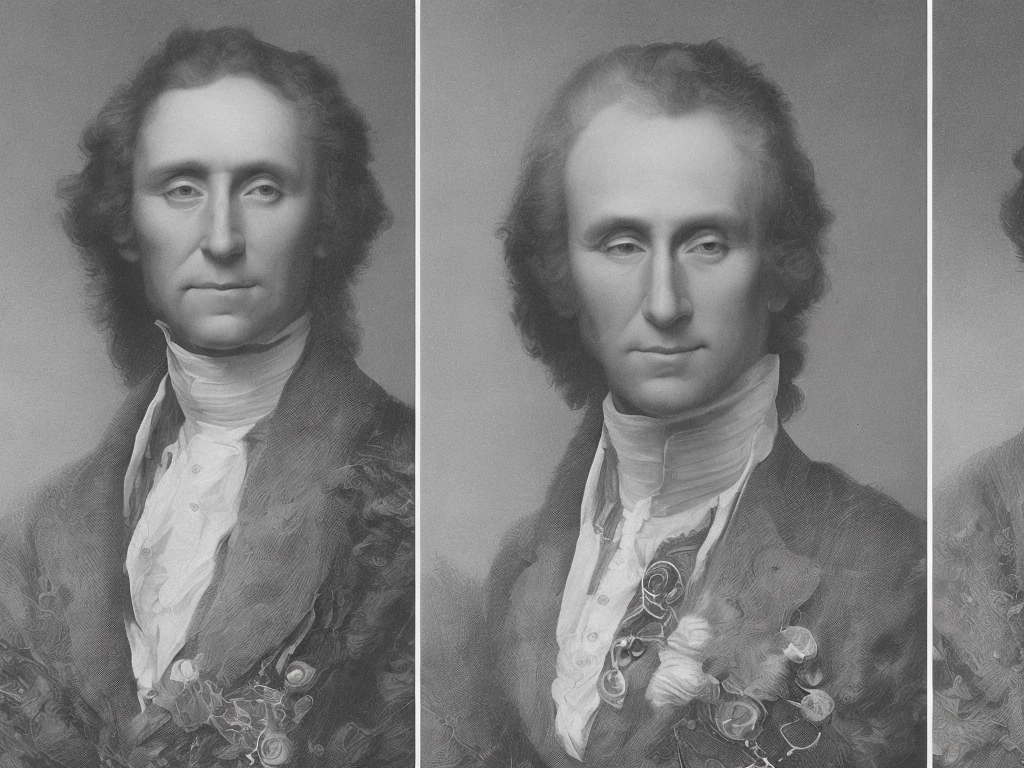# Difference Between 2 Dates

tl;dr
The difference between two dates can be calculated using various methods, such as subtracting the years, months, and days individually or converting both dates to a common unit (usually days) and adjusting for leap years.The Difference Between Two Dates: Understanding Time Intervals

Time is an essential concept that governs our lives. We measure years, months, days, hours, and minutes to keep track of events, appointments, and deadlines. However, understanding the difference between two dates can sometimes be a challenging task, especially when dealing with irregular intervals or a significant time span. In this article, we will explain the different methods used to calculate the difference between two dates, and discuss the challenges and solutions associated with each method.

Before diving into the calculations, it is important to understand the basics of the different units of time measurement. The largest unit is the year, followed by the month, day, hour, minute, and second. While years and months can vary in length, days, hours, minutes, and seconds are consistent. For the purpose of this article, we will focus on the difference between two dates in terms of years, months, and days.

The simplest method to calculate the difference between two dates is by considering each unit of time individually and using basic subtraction. For example, if we have two dates, such as April 6, 2022, and November 15, 2023, we can start by subtracting the years: 2023 - 2022 = 1 year. Next, we subtract the months, taking into consideration that there are 12 months in a year: 11 - 4 = 7 months. Finally, we subtract the days: 15 - 6 = 9 days. The result is a time interval of 1 year, 7 months, and 9 days between the two dates.

While this method provides a quick and easy way to calculate the difference between two dates, it may not always be accurate due to inconsistencies in month lengths. For instance, specific months differ in the number of days: February has 28 or 29 days in a leap year, while the others have 30 or 31. If the dates fall within different months, we need to consider the varying lengths of those months to obtain an accurate result. For example, if we have two dates: May 25, 2022, and June 5, 2023, we might instinctively estimate the difference to be a little over a year. However, using the subtraction method, we would obtain a result of 0 years, -7 months, and -20 days, which is incorrect. To solve this, we need to adjust our calculation by considering the number of days in each month. In this case, May has 31 days, and June has 30 days, making the actual difference 1 year, 0 months, and 11 days.

To overcome the challenges associated with varying month lengths, another method to calculate the difference between two dates is by converting them to a common unit of time, such as days. By converting both dates to the number of days since a reference point, usually January 1, 0000, we can simply subtract these values to obtain the time interval. This method eliminates the complications caused by different month lengths since all months are assumed to have 30 days.

However, this method still presents challenges when dealing with leap years. A leap year occurs every four years, with the exception of years that are divisible by 100 but not divisible by 400. This adjustment accounts for the fact that the Earth's orbit around the sun is not precisely 365 days long. If we use the conversion method on two dates that fall in different leap years, we have to consider the additional day in the leap year.

For example, if we have two dates: February 28, 2020, and February 29, 2024, converting them to days using the conversion method would give us 736,423 days and 738,129 days respectively. By subtracting these values, we obtain a time interval of 1,706 days. This method takes into account the leap year in 2024, resulting in a more accurate calculation.

It is important to remember that these methods provide an approximate value for the time interval, as they do not account for smaller units of time such as hours, minutes, and seconds. To obtain a more precise calculation, one must use more complex calculations that consider these smaller units.

In conclusion, the difference between two dates can be calculated using various methods. The simplest method involves subtracting the years, months, and days individually, which is quick but may not account for varying month lengths. Another method involves converting both dates to a common unit, usually days, which simplifies the calculations but may still require adjustments for leap years. These methods provide approximate values for time intervals and do not consider smaller units of time. To obtain a more precise calculation, more complex calculations are required. Regardless of the method used, understanding the difference between two dates is essential in various fields such as finance, project management, and personal scheduling.